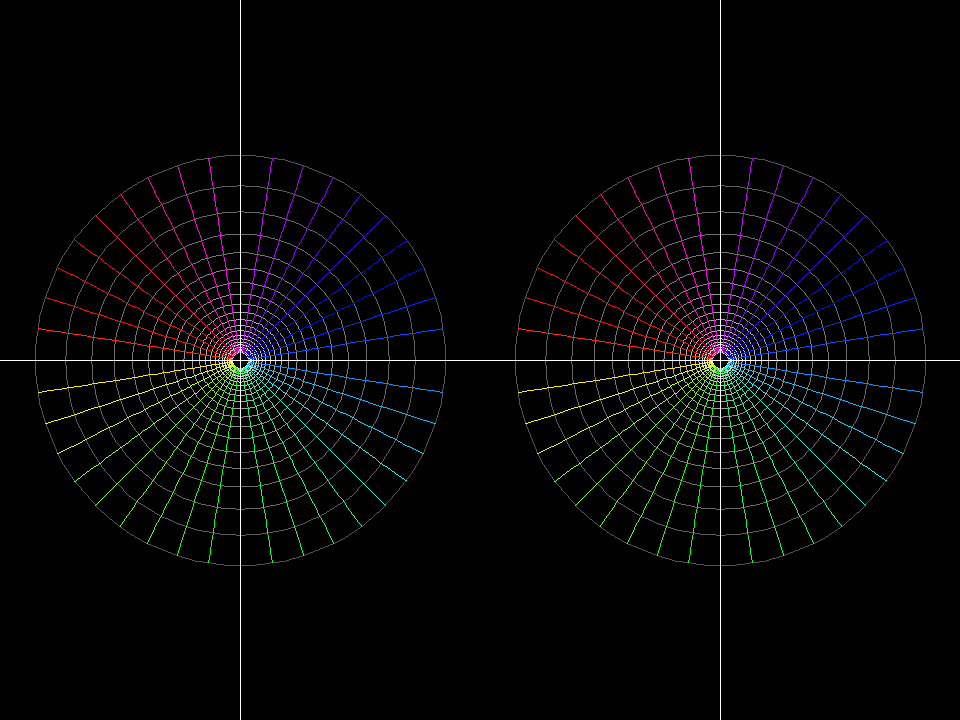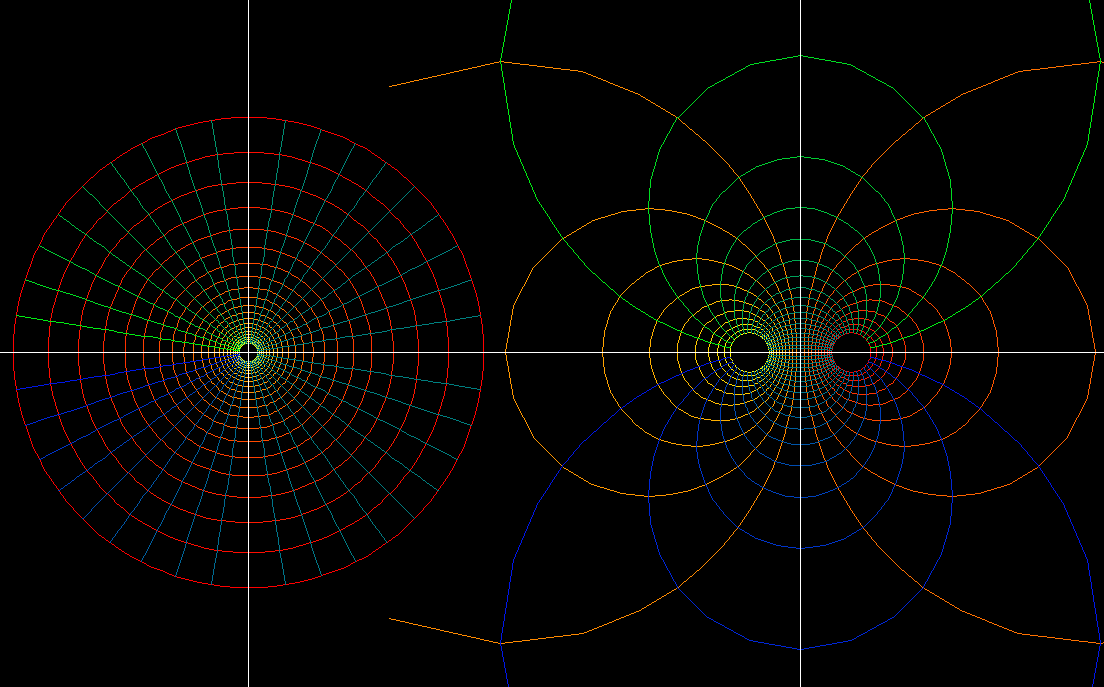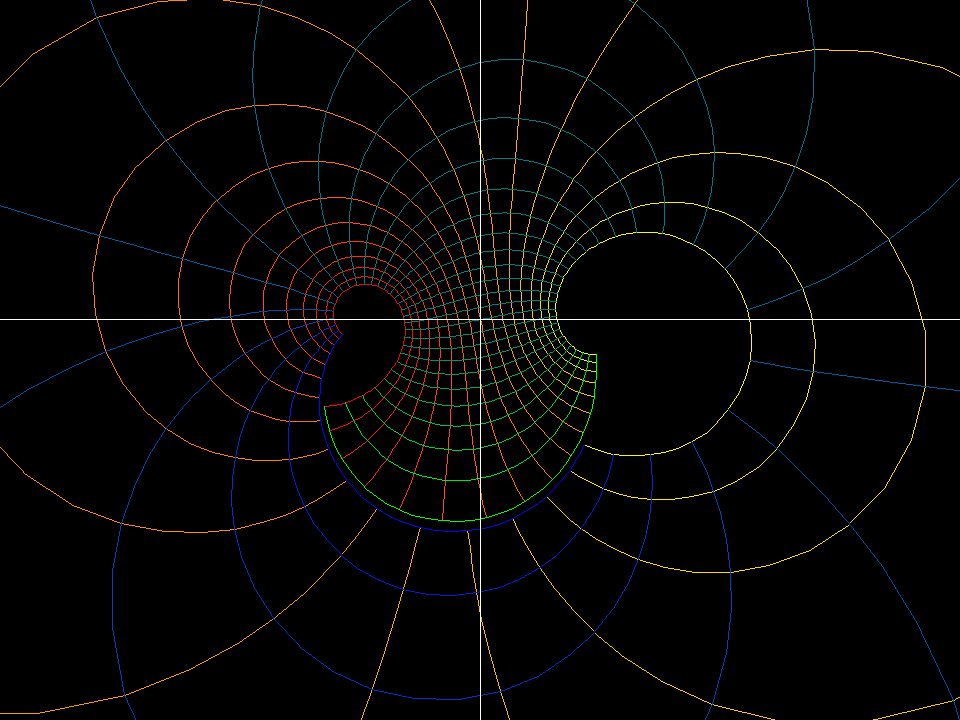# Mobius Transformation

A Mobius Transformation (aka fractional linear functions) is this function:

`f(z) := (a z+b)/(c z+d)`

They differ from the inverse function `1/z` [see Complex Inversion] by adding translation, rotation and scaling.

Because (for c ≠ 0)

```(az+b)/(cz+d) = a/c * (cz + bc/a)/(cz + d) = a/c*(cz + d )/(cz + d) + (b - ad/c)/(cz + d)```

so that

`(az+b)/(cz+d) = a/c + (bc - ad)/c^2 * 1/(z + d/c)`.

This implies that arbitrary Moebius transformations are not more complicated than f(z) = 1/z. In particular: all Moebius transformations map lines and circles to lines and circles, or just circles to circles when stereographically projected to the sphere.function: f(z) = (1+a)*z/(a*z + 1) domain: polar grid 1/5 < |z| < 5 morph: 0 < a < 1moebius riemann 001 map: z ⟶ f(z) = (1+a)*z/(z + a) domain: polar grid 1/4 < |z| < 4 morph: 0 < a < 1 range: Riemann Sphere, anaglyph.moebius rotate 001
• function: `f(z) = (exp(i*c) *z - 1)/(exp(i*c) *z + 1)`
• domain: polar grid `1/5 < |z| < 5`
• range: Gaussian plane
• morph: `0 < c < 2*pi`

All these moebius transformations map 0 to -1 and infinity to +1. We can view the changing grid lines as “moebius rotation” around -1 and +1. The name is justified because these maps are true rotations when viewed on the Riemann sphere. The inverse function f(z) = 1/z is, on the Riemann sphere, 180 degree rotation around -1, +1. The changing grid lines therefore represent a homotopy from the identity to the inverse function and back to the identity.The previous moebius transformations, viewed on the Riemann sphere, are rotations around the x-axis.

## LoxodromicLoxodromic Moebius transformations with fixed points zero and infinity. A spiral grid is mapped to itsself.Loxodromic Moebius transformation with fixed points -1, +1. Apply z ⟶ (1 - z)/ (1 + z) to the previous images.

z_mobius_transformations.pdf